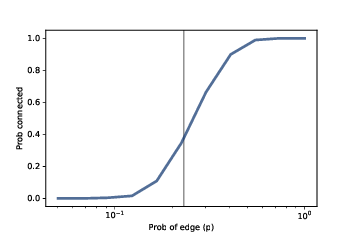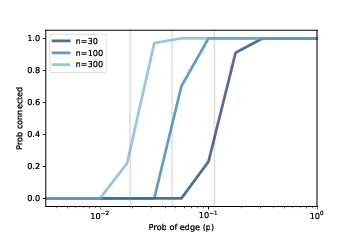# 4.8. Probability of Connectivity¶Figure 4.5: Probability of connectivity with n=10 and a range of p. The vertical line shows the predicted critical value.¶Figure 4.6: Probability of connectivity for several values of n and a range of p.¶

For given values of $$n$$ and $$p$$, we would like to know the probability that $$G(n, p)$$ is connected. We can estimate it by generating a large number of random graphs and counting how many are connected. Here’s how:

def prob_connected(n, p, iters=100):
tf = [is_connected(make_random_graph(n, p))
for i in range(iters)]
return np.mean(bool)


The parameters n and p are passed along to make_random_graph; iters is the number of random graphs we generate.

The result, tf, is a list of boolean values: True for each graph that’s connected and False for each one that’s not.

np.mean is a NumPy function that computes the mean of this list, treating True as 1 and False as 0. The result is the fraction of random graphs that are connected.

>>> prob_connected(10, 0.23, iters=10000)
0.33


We chose 0.23 because it is close to the critical value where the probability of connectivity goes from near 0 to near 1. According to Erdős and Rényi, $$p* = lnn / n = 0.23$$.

We can get a clearer view of the transition by estimating the probability of connectivity for a range of values of $$p$$:

n = 10
ps = np.logspace(-2.5, 0, 11)
ys = [prob_connected(n, p) for p in ps]


The NumPy function logspace returns an array of $$11$$ values from $$10^{−2.5}$$ to $$10^0 = 1$$, equally spaced on a logarithmic scale.

For each value of p in the array, we compute the probability that a graph with parameter p is connected and store the results in ys.

Figure 4.5 shows the results, with a vertical line at the computed critical value, $$p* = 0.23$$. As expected, the transition from 0 to 1 occurs near the critical value.

Figure 4.6 shows similar results for larger values of n. As n increases, the critical value gets smaller and the transition gets more abrupt.

These experimental results are consistent with the analytic results Erdős and Rényi presented in their papers.

You have attempted of activities on this page